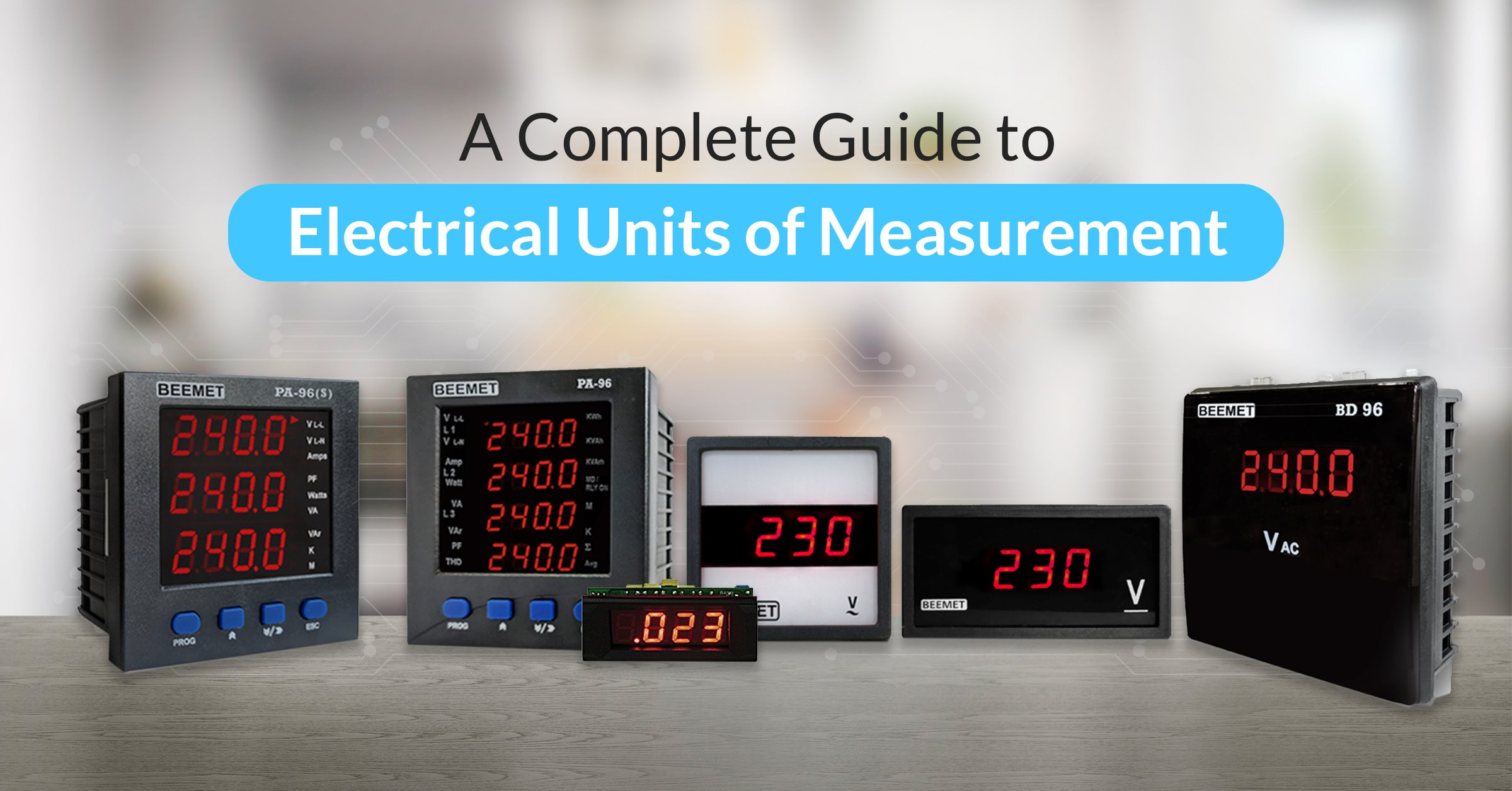# A Complete Guide to Electrical Units of Measurement

#### Dr. Arvind Kulkarni

Dr. Arvind Kulkarni is a seasoned expert in electrical instrumentation with over a decade of experience. Holding a Ph.D. from one of India’s top institutes in Electrical Engineering, he combines academic excellence with practical expertise to deliver precise instruments like Ammeters, Voltmeters, Panel meters, and Current Transformers that you can trust.

In addition to his Ph.D., Dr. Kulkarni also holds prestigious electrical instrumentation and measurement certifications, making him a trusted authority in the industry.

The electrical units of measurement of electrical expressions are based on the International System (SI) of units. The other units are derived from this SI unit of electricity. These units are used in electrical circuits, electrical appliances and other electronics to measure and describe their capacity from small to large.

## Fundamental Units of Electricity

• Voltage

Voltage symbolises the amount of work required to transfer one electric charge from one location to another. It is measured in Volts (V)

• Current

Current refers to the amount of charge that flows across the circuit in a single unit of time. It is measured in Ampere (A).

• Resistance

Resistance refers to the opposition offered by the current flow in the circuit. It is measured in terms of Ohms (Ω).

• Power

Power refers to the product of work done and the number of electrons travelling through a circuit in a single unit of time. It is measured in terms of Watts (W).

## Standard Electric Units of Measure

The standard electrical units of measure are as follows:

 Electrical Parameter Measuring Unit Unit/Symbol Formula Voltage Volt V or E V= I x R Current Ampere I or i I= V/R Resistance Ohm R or Ω R= V/I Charge Coulomb C Q= C x V Power Watts W P= V x I Or I(2) x R Capacitance Farad C C= Q/ V Conductance Siemen G G= 1/R Inductance Henry L/H VL= -L(di/dt) Frequency Hertz Hz f= 1/T Impedance Ohm Z Z(2)= R(2) + X(2)

## Multiples & Sub-Multiples

There is a vast range of electrical values between a minimum and maximum value of a standard electrical unit. For instance, the resistance of a conductor can be as low as 0.001 ohms or as high as 100000 ohms. To avoid writing multiple zeros while describing the values of the electrical unit, we can take the help of the sub-multiples and multiple values of the standard unit.

 Prefix Symbol Multiplier Power of Ten pico 1/1,000,000,000,000 p 10 (12) nano 1/1,000,000,000 n 10 (9) micro 1/1,000,000 μ 10 (6) mini 1/1,000 m 10 (3) centi 1/1,00 c 10 (2) none 1 none 10 (0) kilo 100 c 10 (-2) kilo 1,000 K 10 (-3) Mega 1,000,000 M 10 (-6) Giga 1,000,000,000 G 10 (-9)

## Other Electrical Units of Measurement

Apart from the standard electrical units of measure noted above, there are a bunch of other units too that are used in electrical engineering to denote other values and quantities as follows.

• Watt-Hour (Wh): It refers to the amount of electrical energy consumed by a circuit over a period of time. For instance, a light bulb consumes one hundred watts of electrical power for one hour. Also, Wh is equal to 100 watt-hours, kWh (kilowatt-hour) is equal to 1000 watt-hours, and MWh (megawatt-hours) is equal to 1,000,000 watt-hours.

• Decibel (dB): It is the one-tenth unit of the Bel (B) and it represents the gain in either current, voltage, or power. Being a logarithmic unit, it represents the ratio of input to output in amplifiers, loudspeaker systems, or audio circuits.

• Phase Angle (θ): It is the difference in degrees between the voltage waveform and the current having the same periodic. Moreover, the phase angle of a waveform is measured in degrees or radians.

• Angular Frequency (ω): Angular Frequency is mostly used in a.c. circuits. It represents the Phasor Relationship between two or more waveforms. Moreover, it is a rotational unit of angular frequency 2πf with units in radians per second. The complete revolution on a single cycle is 360 degrees or 2π and thus a revolution is given as 180 degrees or π rad.

• Time Constant (T): The Time Constant of an impedance circuit is the time it takes for the output to reach 63.7% of its maximum or minimum output value when subjected to a Step Response input.

## FAQs

The multiples and sub-multiples of standard electrical units are used to avoid writing multiple zeros while describing the values of electrical units. They include prefixes such as pico (p), nano (n), micro (μ), milli (m), centi (c), kilo (k), mega (M), and giga (G), each with a corresponding multiplier and power of ten.

Decibel is a logarithmic unit that represents the ratio of input to output in amplifiers, loudspeaker systems, or audio circuits. It is used to compare the relative strength of different signals, such as in an audio system.

A watt-hour (Wh) refers to the amount of electrical energy consumed by a circuit over time, while a kilowatt-hour (kWh) is equal to 1000 watt-hours. For example, a 100-watt light bulb consumed for one hour would be 100 watt hours, or 0.1 kilowatt-hours.